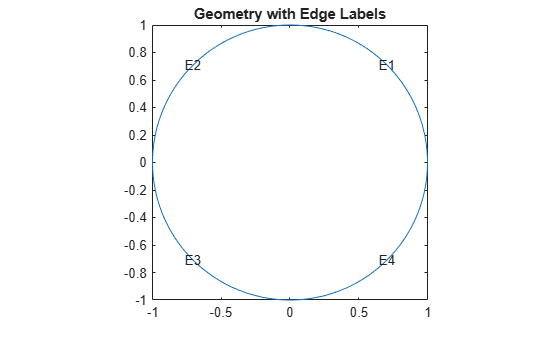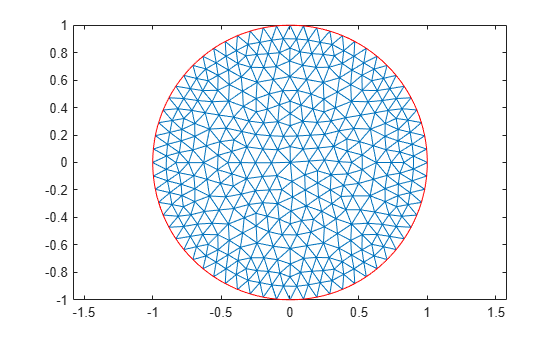Documentation

### This is machine translation

Mouseover text to see original. Click the button below to return to the English version of the page.

To view all translated materials including this page, select Country from the country navigator on the bottom of this page.

## Minimal Surface Problem

This example shows how to solve the minimal surface equation

`$-\nabla \cdot \left(\frac{1}{\sqrt{1+{|\nabla u|}^{2}}}\nabla u\right)=0$`

on the unit disk $\Omega =\left\{\left(\mathit{x},\mathit{y}\right)|{\mathit{x}}^{2}+{\mathit{y}}^{2}\le 1\right\}$, with $u\left(x,y\right)={x}^{2}$ on the boundary $\partial \Omega$.

This example uses the programmatic workflow. For the PDE Modeler app solution, see Minimal Surface Problem: PDE Modeler App.

An elliptic equation in the toolbox form is

`$-\nabla \cdot \left(c\nabla u\right)+au=f.$`

Therefore, for the minimal surface problem, the coefficients are as follows:

`$c=\frac{1}{\sqrt{1+{|\nabla u|}^{2}}},\phantom{\rule{1em}{0ex}}a=0,\phantom{\rule{1em}{0ex}}f=0.$`

Because the coefficient $c$ is a function of the solution $u$, the minimal surface problem is a nonlinear elliptic problem.

To solve the minimal surface problem using the programmatic workflow, first create a PDE model with a single dependent variable.

```numberOfPDE = 1; model = createpde(numberOfPDE);```

Create the geometry and include it in the model. The `circleg` function represents this geometry.

`geometryFromEdges(model,@circleg);`

Plot the geometry displaying the edge labels.

```pdegplot(model,'EdgeLabels','on'); axis equal title 'Geometry with Edge Labels';```Specify the coefficients.

```a = 0; f = 0; cCoef = @(region,state) 1./sqrt(1+state.ux.^2 + state.uy.^2); specifyCoefficients(model,'m',0,'d',0,'c',cCoef,'a',a,'f',f);```

Specify the boundary conditions using the function $u\left(x,y\right)={x}^{2}$.

```bcMatrix = @(region,~)region.x.^2; applyBoundaryCondition(model,'dirichlet',... 'Edge',1:model.Geometry.NumEdges,... 'u',bcMatrix);```

Generate a mesh.

```generateMesh(model,'Hmax',0.1); figure; pdemesh(model); axis equal```Solve the problem by using the `solvepde` function. Because the problem is nonlinear, `solvepde` invokes a nonlinear solver. Observe the solver progress by setting the `SolverOptions.ReportStatistics` property of the model to `'on'`.

```model.SolverOptions.ReportStatistics = 'on'; result = solvepde(model);```
```Iteration Residual Step size Jacobian: Full 0 1.8540e-02 1 2.8715e-04 1.0000000 2 1.2144e-06 1.0000000 ```
`u = result.NodalSolution;`

Plot the solution.

```figure; pdeplot(model,'XYData',u,'ZData',u); xlabel 'x' ylabel 'y' zlabel 'u(x,y)' title 'Minimal Surface'```##### SupportGet trial now